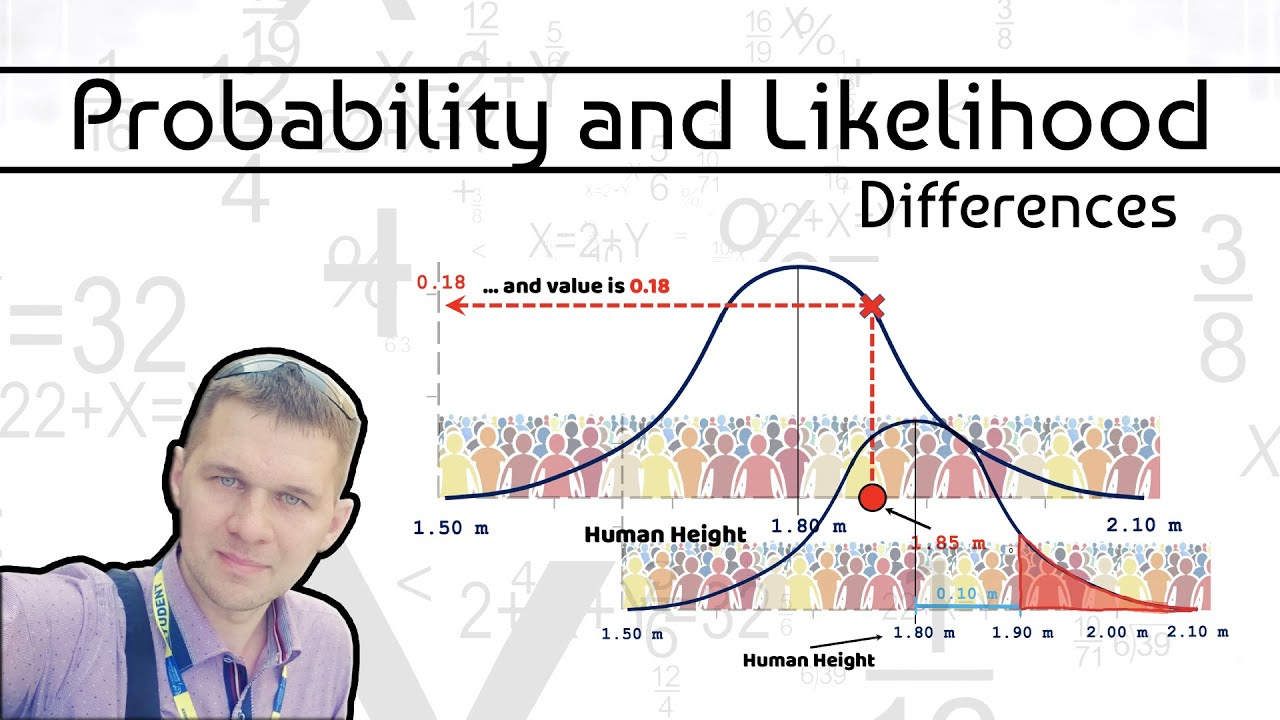1625729040

# Probability and Likelihood. (Differences)

Probability and Likelihood in statistics and math world can be mixed very often. In real life situations most of us (I guess) do not see differences in such mathematics domains. Me too, until I analyzed couples of examples I based this explanatory video on for you.

So:
0:27 #Probability: Area under the curve given by selected criteria with defined distribution of samples having constant mean and standard deviation

3:05 #Likelihood: Y-axis values for fixed data points with distributions that can be moved.

In this video I use the case with randomly selected human heights as an statistical example.

Statistics is easy and fun - enjoy learning!

#statistics
#datasciencetips
#probability

#statistics #probability